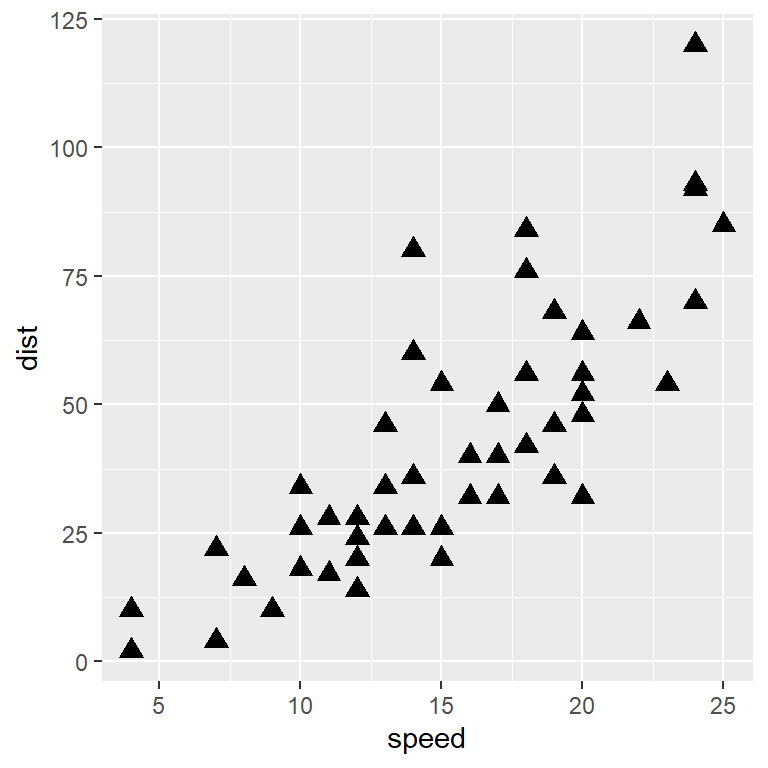# Scatter plot in ggplot2

## Scatter plot in ggplot2 with `geom_point`

The `geom_point` function can be used to create a basic scatter plot in ggplot2. Considering the `cars` data set you can create the following visualization.

``````# install.packages("ggplot2")
library(ggplot2)

# Basic scatter plot
ggplot(cars, aes(x = speed, y = dist)) +
geom_point()``````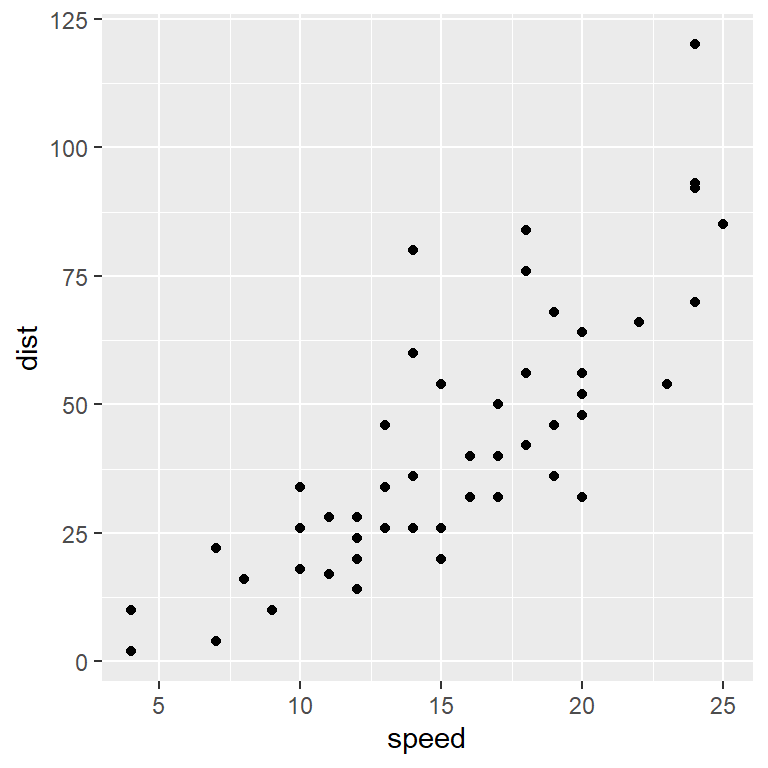### Color customization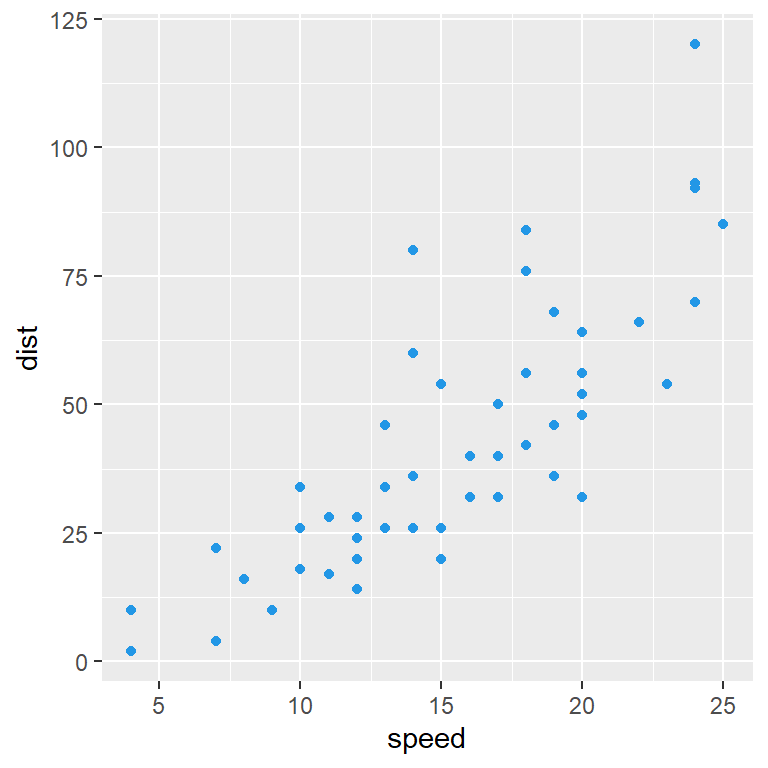Color

The `colour` argument of the `geom_point` function allows customizing the color or the points.

``````# install.packages("ggplot2")
library(ggplot2)

ggplot(cars, aes(x = speed, y = dist)) +
geom_point(colour = 4)``````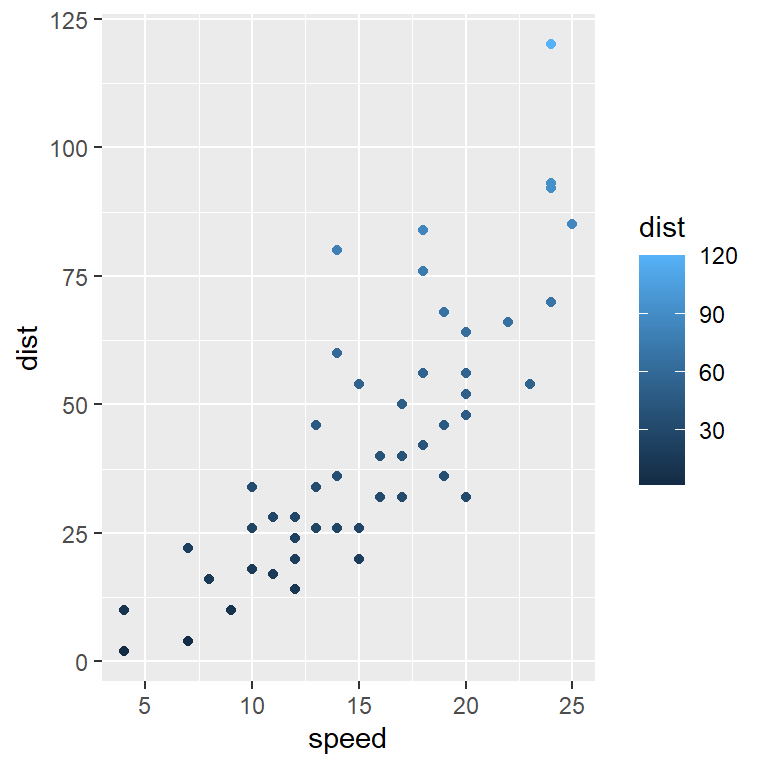If you want to colorize the points based on a value you can pass the variable to the `color` (`colour`) argument of `aes`.

``````# install.packages("ggplot2")
library(ggplot2)

ggplot(cars, aes(x = speed, y = dist, color = dist)) +
geom_point()``````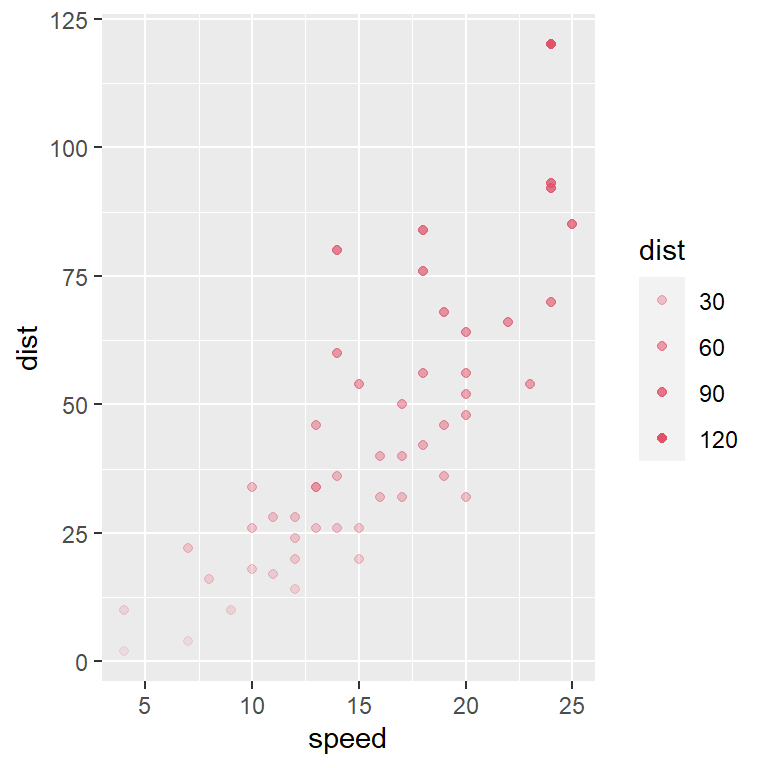Transparency

The transparency of the points can also be customized with `alpha` argument. You can pass a value or a variable, so the transparency will be based on that variable, as in the example below.

``````# install.packages("ggplot2")
library(ggplot2)

ggplot(cars, aes(x = speed, y = dist, alpha = dist)) +
geom_point(colour = 2)``````

Setting `show.legend = FALSE` inside `geom_point` will remove the legends created automatically when you specify the `colour` or `alpha` aesthetics or both.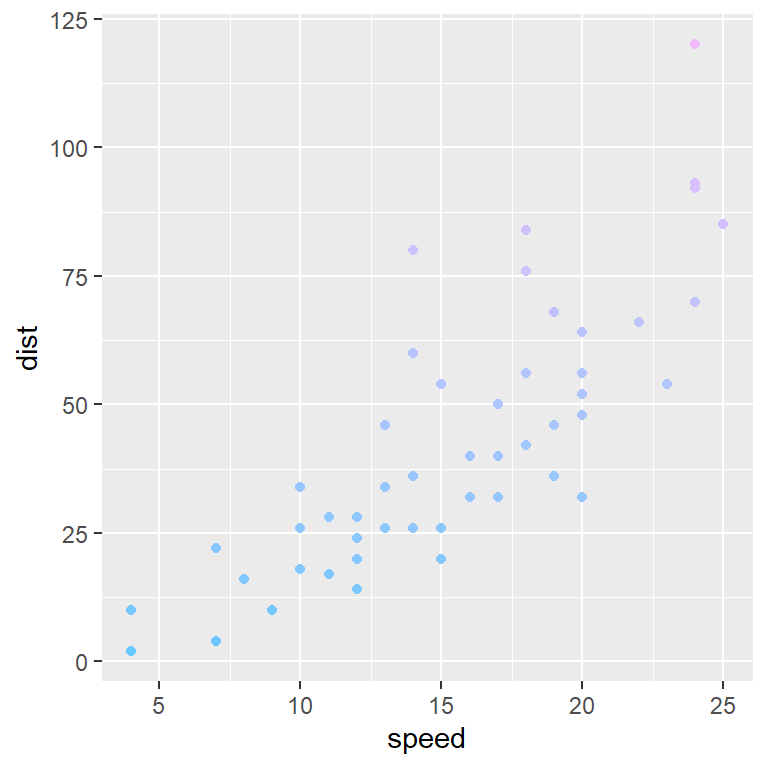Color scale

If you pass a variable to the `colour` argument of the `aes` you can customize the color scale with `scale_color_gradient` (or a similar function), setting the lower and the higher color value.

``````# install.packages("ggplot2")
library(ggplot2)

ggplot(cars, aes(x = speed, y = dist,
colour = dist)) +
geom_point(show.legend = FALSE) +
scale_color_gradient(low = "#67c9ff", high = "#f2bbfc")``````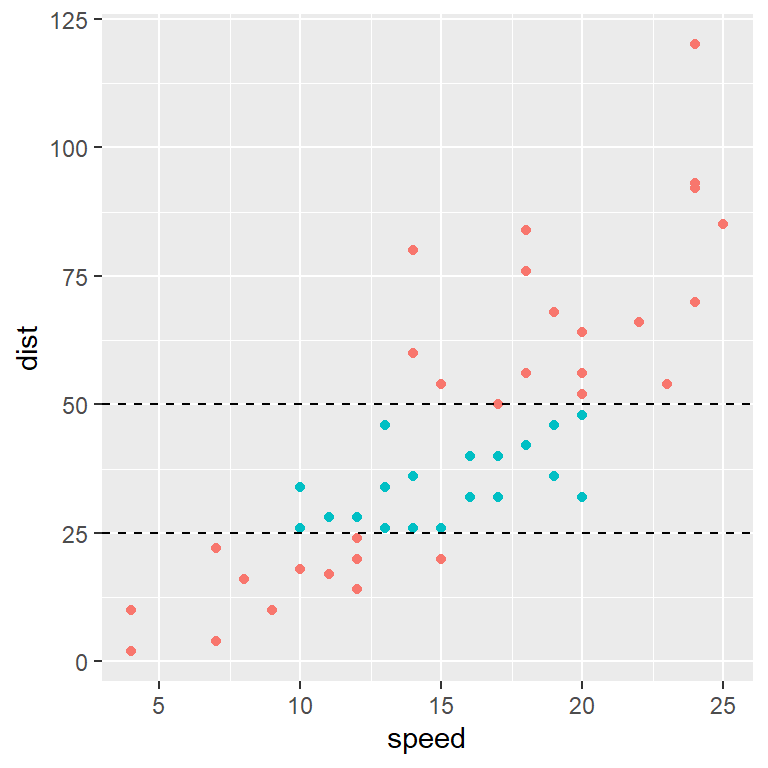Color based on values

The `geom_point` function allows coloring the points based on some conditions. Note that you can customize the color of the points with `scale_color_manual`.

``````# install.packages("ggplot2")
library(ggplot2)

ggplot(cars, aes(x = speed, y = dist)) +
geom_point(aes(colour = dist > 25 & dist < 50),
show.legend = FALSE) +
geom_hline(yintercept = 25, linetype = "dashed") +
geom_hline(yintercept = 50, linetype = "dashed")``````

### Shape and size

Shape and size of the points

The shape and the size of the points can be changed with `size` and `shape` arguments, respectively.

``````# install.packages("ggplot2")
library(ggplot2)

ggplot(cars, aes(x = speed, y = dist)) +
geom_point(size = 3, shape = 17)``````